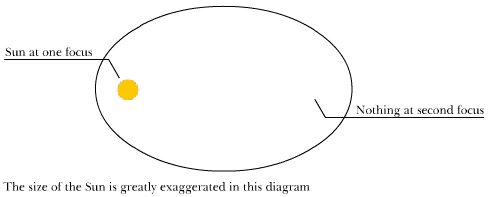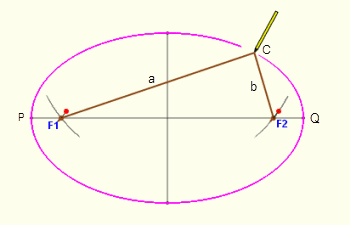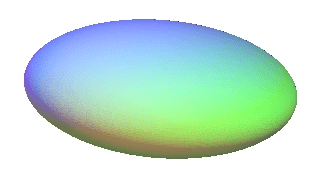# Reconciling different ways to define an ellipse

• B
• DaveC426913

#### DaveC426913

Gold Member
TL;DR Summary
How does an ellipse defined by two foci relate to an ellipse defined by one focus?
An ellipse is a conic section - a slice through a cone. The axis of the cone coincides with the focus of the ellipse.(Correct?)But ellipses can have two foci, not just one, as shown by this method of construction:Questions:
1. Is F1 in the diagram the same as the axis of the conic section?
2. Does F2 have any correlation in the conic model?
3. Is there some way that these two apparently unrelated definitions of an ellipses are two sides of the same coin?

Are there corresponding constructions for ellipses in 3D (i.e. prolate spheroids)?i.e. Can one construct a prolate spheroid with two foci?

(Intuitively, the answer is yes, and quite simply, by merely adding the third variable to the equation)

I didn't realize the focus of an ellipse is the axis of the cone, cool fact.
If you take a fixed plane, you can take an angled cone and intersect it with the plane to make an ellipse. If you reflect the cone, you can get the same ellipse with that other cone. I think that second cone gives you the second focus.

There's a very good video from 3B1B proving the equivalence of the two ellipse definitions:

•••JD_PM, FactChecker and DaveC426913
An ellipse can not be defined only by one focus. There must be additional information to define an ellipse and that is also enough to determine the second focus. The solar system is an example of this. The Sun is a single focus, yet there are many planets orbiting it along different elliptical paths. So the next question is what additional information are you willing to specify? We need to know that before we can answer how the second focus can be determined.

•mfb
I see that you are asking if the definition of "focus" is the same for both definitions of an ellipse. Do you have a reference that defines "focus" and "axis" as you are using it?
The axis of a cone is through the center of the cone and it does not coincide with the Sun's location.

An ellipse can not be defined only by one focus.
Yes. I should have said 'has a only single focus as part if its definition'.

The axis of a cone is through the center of the cone and it does not coincide with the Sun's location.
Indeed. My guess was wrong.

I didn't realize the focus of an ellipse is the axis of the cone, cool fact.

Alas. T'isn't. Check out the excellent video posted by @Gaussian97 , above, at timestamp 4:17.

•FactChecker
Thanks guys.
Esp. @Gaussian97 for answering the question in one swell foop.

Yes. I should have said 'has a only single focus as part if its definition'.

Indeed. My guess was wrong.

Alas. T'isn't. Check out the excellent video posted by @Gaussian97 , above, at timestamp 4:17.
A little wrong, but still a good question. And the link given by @Gaussian97 is excellent.

If you take a fixed plane, you can take an angled cone and intersect it with the plane to make an ellipse.
In fact, if you intersect a cone with various planes, at different angles, you get all of the conic sections. Keep in mind that, mathematically, a cone is the surface swept out by two lines that intersect, that are rotated about a common axis. So, mathematically, a cone has an upper portion and a lower portion, with both meeting at the point where the two lines intersect.

If the intersecting plane is perpendicular to the axis of the cone, you get a circle.
If the intersecting plane is parallel to one of the cone-generating lines, you get a parabola.
If the intersecting plane is parallel to the central axis, you get the two sheets of a hyperbola.
If the intersecting plane meets none of the above criteria, you get an ellipse.
It's also worth noting that a circle is sort of a degenerate ellipse, one in which the two foci are at the same point.

If the intersecting plane is parallel to the central axis, you get the two sheets of a hyperbola.
If the intersecting plane meets none of the above criteria, you get an ellipse.
For a hyperbola it doesn't need to be parallel to the central axis. It just needs to be within the opening angle, i.e. cross both halves of the ellipse.
i.e. Can one construct a prolate spheroid with two foci?
Yes, it's just the rotation of the ellipse around its semimajor axis. It should also be the intersection of a 4D cone with a 3D hyperplane.

For a hyperbola it doesn't need to be parallel to the central axis. It just needs to be within the opening angle, i.e. cross both halves of the ellipse.
Yes, it's just the rotation of the ellipse around its semimajor axis.
Right. My description was a bit too restrictive.

Yes, it's just the rotation of the ellipse around its semimajor axis. It should also be the intersection of a 4D cone with a 3D hyperplane.
Yes, but you're sort of switching construction techniques there.

I wondered if one could use the same thumbtacks and string method.

OK, yes it's trivially possible to construct an arbitrarily large number of 2D ellipses in an arbitrarily large number of planes all rotated about the axis, but what about a construction that has full 3D freedom of motion, not a procedural buildup of discrete ellipses?

Thinking...thinking...

Yes. it's the same thing. A prolate spheroid could be drawn by giving the pencil complete (even random) freedom of movement in all 3 physical planes simultaneously - constrained only by the string.

And that's pretty much what you were saying. Cool. You could draw arbitrarily short arcs of an arbitrary number of 2D ellipses, each in its own plane, interspersed with arbitrarily short rotations about the semi-major axis of the 3D spheroid.

Last edited:
•hutchphd